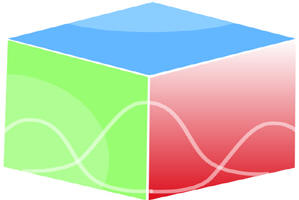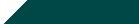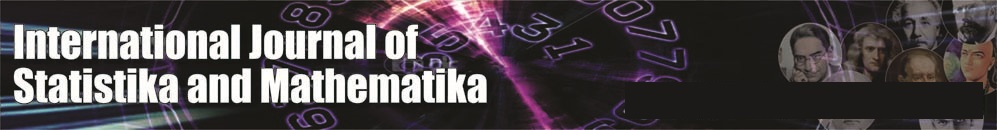``Home| Journals | Statistics Online Expert | About Us | Contact UsUntitled Document

[

Benefit-benefit analysis of a two-dissimiliar cold standby system subject to rainfall and switch failure with general as well as Erlang-k repair facility as first come last serve

Ashok Kumar Saini

Associate Professor, Department of Mathematics, BLJS College, Tosham, Bhiwani, Haryana, INDIA.

Email: [email protected]

Research Article

Abstract               Introduction: To transfer a unit from the standby state to the online state, a device known as ‘switching device’ is required. Generally, we assume that 1 the switching device is perfect in the sense that it does not fail and 2 the repair facility is first come first serve basis.. However, there are practical situations where the switching device can also fail and the repair facility is FCLS i.e. First Come Last Serve. We have taken two dissimilar units failure due to rainfall and switch failure distribution as exponential and repair time distribution as General as well as Erlang-K phase type. We have find out MTSF, Availability analysis, the expected busy period of the server for repair the failed unit under rainfall in (0,t], expected busy period of the server for repair in(0,t], the expected busy period of the server for repair of switch failure in (0,t], the expected number of visits by the repairman for failure of units in (0,t],the expected number of visits by the repairman for switch failure in (0,t] and cost- benefit analysis using regenerative point technique. A special case using failure and repair distributions as exponential is derived and graphs have been drawn.

Keywords: Cold Standby, rainfall, First Come Last Serve, MTSF, Availability, Busy period, cost-benefit Function.

INTRODUCTION

To transfer a unit from the standby state to the online state, a device known as ‘switching device’ is required. Generally, we assume that the switching device is perfect in the sense that it does not fail. However, there are practical situations where the switching device can also fail. This has been pointed out by Gnedenko et al (1969).Such system in which the switching device can fail are called systems with imperfect switch. In the study of redundant systems it is generally assumed that when the unit operating online fails, the unit in standby is automatically switched online and the switchover from standby state to online state is instantaneous. In this paper, we have RAINFALL which are non-instantaneous in nature. We assume that the RAINFALL cannot occur simultaneously in both the units and when there occurs RAINFALL of the non –instantaneous nature the operation of the unit stop automatically. Here, we investigate a two-unit (non-identical) cold standby –a system in which offline unit cannot fail with switch failure under the influence of RAINFALL. The RAINFALL cannot occur simultaneously in both the units and when there are less RAINFALL, that is, within specified limit the unit operates as normal as before. But if these are beyond the specified limit the operation of the unit is stopped to avoid excessive damage of the units and as the RAINFALL goes on some characteristics of the stopped unit change which we call failure of the unit. After the RAINFALL are over the failed unit undergoes repair immediately according to first come last served discipline. For example, when a train came on the junction Station it waits for crossing of other train. When the other train came it stops and departs first according to FCLS.

ASSUMPTIONS

The system consists of two dissimilar cold standby units and the rainfall and failure time distribution are exponential with rates λ1, λand λ3 whereas the repairing rates for repairing the failed system due to rainfall and due to switch failure are arbitrary with CDF G(t) and G(t) respectively.

1. The operation of units stops automatically when rainfall occurs so that excessive damage of the unit can be prevented.
2. The rainfall actually failed the units. The rainfall are non-instantaneous in nature and it cannot occur simultaneously in both the units.
3. The repair facility works on the first come last serve (FCLS) basis.
4. The switches are imperfect and instantaneous.
5. All random variables are mutually independent.

SYMBOLS FOR STATES OF THE SYSTEM

Superscripts: O, CS, SO, F, SFO

Operative, cold Standby, Stops the operation, Failed, Switch failed but operable respectively

Subscripts: nf, uf,ur, wr, uR

No rainfall, under rainfall, under repair, waiting for repair, under repair continued respectively

Up states: 0,1,2,6; Down states: 3,4,5,7,8

STATES OF THE SYSTEM

0(Onf, CSnf)

One unit is operative and the other unit is cold standby and there are no rainfall in both the units.

1(SOnf, Onf)

The operation of the first unit stops automatically due to rainfall and cold standby units starts operating.

2(SOnf, SFOnf,ur)

The operation of the first unit stops automatically due to rainfall and during switchover to the second unit switch fails and undergoes repair.

3(Fur, Ouf)

The first one unit fails and undergoes repair after the rainfall are over and the other unit continues to be operative with no rainfall.

4(Fur, SOuf)

The one unit fails and undergoes repair after the rainfall are over and the other unit also stops automatically due to rainfall.

5(FuR, Fwr)

The repair of the first unit is continued from state 4 and the other unit is failed due to rainfall in it and is waiting for repair.

6(FuR, SOuf)

The repair of the first unit is continued from state 3 and in the other unit rainfall occur and stops automatically due to rainfall.

7(Fwr, SFOuR)

The repair of failed switch is continued from state 2 and the first unit is failed after rainfall and waiting for repair.

TRANSITION PROBABILITIES

Simple probabilistic considerations yield the following expressions:

p01= , p02 =

p13= , p14=

p23= λ1G2*( λ2), p237= λ2G2*( λ2), p24= 2*( λ2),

p30= G1*( λ1), p336= 1*( λ1)

p43 = G1*( λ2), p435 = G1*( λ2)                                                                                                                                                         (1)

Figure 1: The State Transition Diagram

We can easily verify that

p01 + p02= 1, p13 + p14= 1, p23+ p231 + p24= 1, p30 +p336 = 1,

p43+ P435= 1                                                                                                                                                                                         (2)

And mean sojourn time are

µ0 = E(T) = = 1/ λ1

Similarly

µ1 = 1/ λ2, µ2 = ,

µ4 =                                                                                                                                                                    (3)

MEAN TIME TO SYSTEM FAILURE

We can regard the failed state as absorbing

,                                                                           (4-6)

Taking Laplace-Stieltjes transforms of eq. (4-6) and solving for

= N1(s) / D1(s)                                                                                                                                                              (7)

Where

N1(s) = {  +

D1(s) = 1 -

Making use of relations (1) and (2) it can be shown that  =1, which implies

that  is a proper distribution.

MTSF = E [T] =     = (D1(0) - N1(0)) / D1 (0)

s=0

= ( +p01  + p01 p13 ) / (1 - p01 p13 p30 )                                                                                                                       (8)

where

+ ,  + ,  + (1) + ,,  + (6)

+ (5)

AVAILABILITY ANALYSIS

Let Mi(t) be the probability of the system having started from state i is up at time t without making any other regenerative state belonging to E. By probabilistic arguments, we have

The value of M0 (t) = λ1 λ3t M1 (t)= λ1 λ2t

M3 (t) λ1 1  ).                                                                                                                                                                   (9)

The point wise availability Ai(t) have the following recursive relations

A0(t) = M0(t) + q01(t)[c]A1(t) + q02(t)[c]A2(t)

A1(t) = M1(t) + q13(t)[c]A3(t) + q14(t)[c]A4(t),

A2(t) = {q23(t) + q23(7)(t)}[c]A3(t) +q33(6)(t)[c]A3(t)

A4(t) = {q43(t) + q43(5)(t)[c]A3(t)                                                                                                                                                   (10 -14)

Taking Laplace Transform of eq. (10-14) and solving for

= N2(s) / D2(s)                                                                                                                                                            (15)

Where

N2(s) = (1 - 33(6)(s)) 0(s) +[ 01(s) 1(s) + 13(s) 14(s) 43(s) + 43(5)(s) 02(s){ 23(s)) + 23(1) (s) 24 (s)( 43 (s) + 43(5)(s))}] 3(s)

D2(s) = (1 - 33(6)(s)) - 30(s) 01(s){ 13(s) 14 (s) ( 43(s) 43(5)(s)) }

+ 20(s) 23(s) 23(7)(s) 24(s)( 43(s)) + 43(5)(s) )}]

A0 =  =  =

Using L’ Hospitals rule, we get

A0 =  =                                                                                                                                     (16)

Where

N2 (0) = p30 0(0) + p01 1(0) 3(0) )

D2(0) =  + p01  + p14 + p02(  + p24 )] p30

The expected up time of the system in (0,t] is

(t) = So that                                                                                             (17)

The expected down time of the system in (0,t] is

(t) = t- (t) So that                                                                                                    (18)

The expected busy period of the server for repairing the failed unit under rainfallin (0,t]

R0(t) = q01(t)[c]R1(t) + q02(t)[c]R2(t)

R1(t) = S1(t) + q13(t)[c]R3(t) + q14(t)[c]R4(t),

R2(t) = S2(t) + q23(t)[c]R3(t) + q23(7)(t)[c]R3(t) + q24(t)[c]R4(t)

R3(t) = q30(t)[c]R0(t) +q33(6)(t)[c]R3(t),

R4(t) = S4(t) + (q43(t)+ q43(5)(t)) [c]R3(t)                                                                                                                                    (19-23)

Where

S1(t)= λ1 λ2t, S2(t) = λ1t 2(t), S4(t) = λ1t 1(t)                                                                                            (24)

Taking Laplace Transform of eq. (19-23) and solving for

= N3(s) / D2(s)                                                                                                                                                              (25)

Where

N3(s) = (1 - 33(6)(s)) [ 01(s)( 1(s) + 14(s) 4(s)+ 02(s)( 2(s) + 24(s) 4(s))] andD2(s) is already defined.

In the long run, R0 =                                                                                                                                                     (26)

whereN3(0)= p30[ p01( 1(0) + p14 4(0) ) + p02 ( 2(0) + p24 4(0) ) and D2(0) is already defined.

The expected period of the system under rainfall in (0,t] is

(t) = So that                                                                                                                  (27)

The expected Busy period of the server for repair of dissimilar units by the repairman in (0,t]

B0(t) = q01(t)[c]B1(t) + q02(t)[c]B2(t)

B1(t) = q13(t)[c]B3(t) + q14(t)[c]B4(t),

B2(t) = q23(t)[c] B3(t) + q23(7)(t)[c]B3(t) + q24(t)[c] B4(t)

B3(t) = T3(t)+q30(t)[c] B0(t) + q33(6)(t)[c]B3(t)

B4(t) = T4 (t)+{ q43(t) + q43(5)(t)} [c]B3(t)                                                                                                                                   (28-32)

Where

T3(t) = λ2t 1(t)           T4(t) = λ1t 1(t)                                                                                                                           (33)

Taking Laplace Transform of eq. (28-32) and solving for

= N4(s) / D2(s) (34)

Where

N4(s) = 3(s) [ 01(s) 13(s) 14(s) 43(s) + 43 (5)(s))}+ 02(s) 23(s)

23(7)(s) 24(s) ( 43(s)  + 43 (5)(s))}]+ 4(s) [ 01(s) 44(s) 33(6)(s)

02(s) 24 (s)(1- 33(6)(s)

In steady state, B0 =                                                                                                                                                     (35)

whereN4(0)= 3(0)+ 4(0) { p30(p01p14 + p02 p24) } and D2(0) is already defined.

The expected busy period of the server for repair in (0,t] is

(t) = So that                                                                                                             (36)

The expected Busy period of the server for repair of switch in (o,t]

P0(t) = q01(t)[c]P1(t) + q02(t)[c]P2(t)

P1(t) = q13(t)[c]P3(t) + q14(t)[c]P4(t),

P2(t) = L2 (t)+ q23(t)[c]P3(t) + q23(7)(t)[c]P3(t)+q24(t)[c]P4(t)

P3(t) = q30(t)[c]P0(t) + q33(6)(t)[c]P3(t),

P4(t) = (q43(t)+ q43(5)(t)) [c]P3(t)                                                                                                                                                  (37-41)

Where L2 (t) = λ1t 2(t)

Taking Laplace Transform of eq. (37-41) and solving for

= N5(s) / D2(s)                                                                                                                                                              (43)

Where N5(s) = 02(s ) 2(s) 1 - 33(6)(s)) andD2(s) is defined earlier.

In the long run, P0 =                                                                                                                                                     (44 )

whereN5(0)= p30 p02 2(0) and D2(0) is already defined.

The expected busy period of the server for repairof the switch in (0,t] is

(t) = So that                                                                                                                (45)

The expected number of visits by the repairman for repairing the different units in (0, t]

H0(t) = Q01(t)[s]H1(t) + Q02(t)[s]H2(t)

H1(t) = Q13(t)[s][1+H3(t)] + Q14(t)[s][1+H4(t)],

H2(t) = [Q23(t) +Q23(7)(t)] [s][1+H3(t)] + Q24(t)[s][1+ H4(t)]

H3(t) = Q30(t)[s]H0(t) + Q33(6)(t)[s]H3(t),

H4(t) = (Q43(t)+ Q43(5)(t)) [s]H3(t)                                                                                                                                                                (46-50)

Taking Laplace Transform of eq. (46-50) and solving for

= N6(s) / D3(s)                                                                                                                                                             (51)

Where

N6(s) = (1 – 33(6)*(s) ){ 01(s) 13(s) 14 (s) 02(s) 24(s)

23 (s) 23(7)(s)

D3(s) = (1 - 33(6)*(s)) – 30(s) 01 (s) { 13(s) 14(s) ( 43*(s) 43(5)(s))}

+ 02(s) 23(s) 23(7)(s) 24(s)( 43(s)) + 43(5)(s)) }]

In the long run, H0 =                                                                                                                                                     (52)

Where N6(0)= p30and D’3(0) is already defined.

The expected number of visits by the repairman for repairing the switch in (0, t]

V0 (t) = Q01(t)[s]V1(t) + Q02(t)[s][1+V2(t)]

V1(t) = Q13(t)[s]V3(t) + Q14(t)[s]V4(t),

V2(t) = Q24(t)[s][1+V4(t)] +[ Q23(t) + Q23(7)(t)[s][1+V3(t)]

V3(t) = Q30(t)[s]V0(t) + Q33(6)(t)[s]V3(t)                                                                                                                                    (53-57)

Taking Laplace-Stieltjes transform of eq. (53-57) and solving for

= N7(s) / D4(s)                                                                                                                                                             (58)

Where N7 (s) = (1 – 33(6)*(s) ){ 01(s) 14(s) 43 (s) 02(s) 24(s) +Q* 02(s)( 23 (s) 23(7)(s)

and D4(s) is the same as D3(s)

In the long run, V0 =                                                                                                                                                     (59)

Where N7 (0)= p30[p01 p14 p43 + p02 ] and D’3(0) is already defined.

COST BENEFIT ANALYSIS

The cost-benefit function of the system considering mean up-time, expected busy period of the system under rainfall when the units stops automatically, expected busy period of the server for repair of unit and switch, expected number of visits by the repairman for unit failure, expected number of visits by the repairman for switch failure. The expected total cost-benefit incurred in (0, t] is, C (t) = Expected total revenue in (0,t] - expected total repair cost for switch in (0,t]

• expected total repair cost for repairing the units in (0,t ]
• expected busy period of the system under rainfall when the units automatically stop in (0,t]
• expected number of visits by the repairman for repairing the switch in (0,t]- expected number of visits by the repairman for repairing of the units in (0,t]

The expected total cost per unit time in steady state is

C =  =

= K1A0 - K2P0 - K3B0 - K4R0 - K5V0 - K6H0

Where

K1: revenue per unit up-time,

K2: cost per unit time for which the system is under switch repair

K3: cost per unit time for which the system is under unit repair

K4: cost per unit time for which the system is under rainfall when units automatically stop.

K5: cost per visit by the repairman for which switch repair,

K6: cost per visit by the repairman for units repair.

CONCLUSION

After studying the system, we have analysed graphically that when the failure rate, rainfall rate increases, the MTSF and steady state availability decreases and the cost function decreased as the failure increases.

REFERENCES

1. Gnedanke, B.V., Belyayar, Yu.K. and Soloyer, A.D., Mathematical Methods of Relability Theory,1969 ; Academic Press, New York.
2. Barlow, R.E. and Proschan, F., Mathematical theory of Reliability, 1965; John Wiley, New York.
3. Dhillon, B.S. and Natesen, J, Stochastic Anaysis of outdoor Power Systems in fluctuating environment, Microelectron. Reliab. 1983; 23,867-881.
4. Goel, L.R., Sharma, G.C. and Gupta, Rakesh Cost Analysis of a Two-Unit standby system with different weather conditions, Microelectron. Reliab., 1985; 25, 665-659.
5. Goel, L.R., Sharma G.C. and Gupta Parveen, Stochastic Behaviour and Profit Anaysis of a redundant system with slow switching device, Microelectron Reliab., 1986; 26, 215-219.